November 29, 2018

## Last class I made a mistake in one slide

### I want to correct that mistake nowWith experimental data from the big coil we found the linear model $\text{n_marbles}=A+B\cdot \text{length}$ and we compared it with the formula from Hooke’s Law $\text{force}=K\cdot(L-\text{length})$

## force is the weight of the marbles

Each marble has mass $$m$$. The force points down, so $-m g\cdot\text{n_marbles}=K\cdot(L-\text{length})$ which can be re-written as $\text{n_marbles}=\underbrace{-\frac{K}{m g}\cdot L}_{A} + \underbrace{\frac{K}{m g}}_{B}\cdot\text{length}$

## CGS units

In this case it is easier to use centimeters, grams and seconds

Thus, force is measured in dyne, and length in cm

Looking at Hooke’s law $\text{force}=K\cdot(L-\text{length})$ we can see that $$K$$ is measured in dyne/cm

## Finding $$K$$

Our model is $\text{n_marbles}=\underbrace{-\frac{K}{m g}\cdot L}_{\texttt{coef(model)}} + \underbrace{\frac{K}{m g}}_{\texttt{coef(model)}}\cdot\text{length}$ therefore $K=\texttt{coef(model)}\cdot m\cdot g$

## Marbles weight $$\approx 20\pm 1$$ grams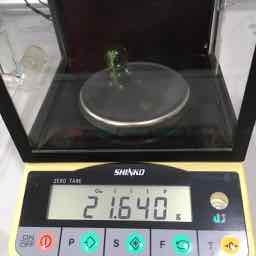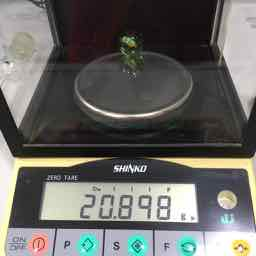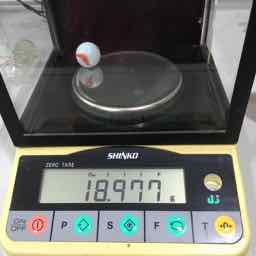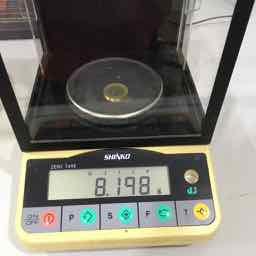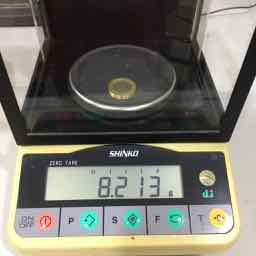## Calculating $$K$$

Taking the mass of marbles as 20gr, we calculate the elasticity constant as

coef(model) * 20 * 9.8
length
37.5 

The units are dyne/cm.

The label length comes from coef(model)

The number is the same as before. The units are correct this time

## Calculating $$L$$

This is the same as last class. Since

$\texttt{coef(model)}=-\frac{K}{m g}L = -\texttt{coef(model)}\cdot L$ we can calculate $$L$$ as $L=-\frac{\texttt{coef(model)}}{\texttt{coef(model)}} = 75.922$

## What is a logarithm?

We need very little math for our course: arithmetic, algebra, and logarithms

If $$x=p^m$$ then $\log_p(x) = m$

If we use another base, for example $$q$$, then $\log_q(x) = m\cdot\log_q(p)$

So if we use different bases, there is only a scale factor

The easiest one is natural logarithm

• They only work with positive numbers. Not with 0
• If $$x=p\cdot q$$ then $\log(x)=\log(p)+\log(q)$
• If $$\log(x)=m$$ then $$x=\exp(m)$$

## Linear models can be used in three cases

Basic linear model $y=A+B\cdot x$ Exponential $y=I\cdot R^x\qquad\log(y)=log(I)+log(R)\cdot x$ Power of $$x$$ $y=C\cdot x^E\qquad\log(y)=log(C)+E\cdot\log(x)$

## Which one to use?

The easiest way to decide is to draw several plots, placing log() in different places, and looking which one seems more like a straight line

For example, let’s analyze data from Kleiber’s Law (Physiological Reviews 1947 27:4, 511-541)

The following data shows a summary. The complete table has 26 animals

## Body size v/s metabolic rate

animal kg kcal
Mouse 0.021 3.6
Rat 0.282 28.1
Guinea pig 0.410 35.1
Rabbit 2.980 167.0
Cat 3.000 152.0
Macaque 4.200 207.0
Dog 6.600 288.0
animal kg kcal
Goat 36.0 800
Chimpanzee 38.0 1090
Sheep ♂ 46.4 1254
Sheep ♀ 46.8 1330
Woman 57.2 1368
Cow 300.0 4221
Young cow 482.0 7754

## First plot: Linear

plot(kcal ~ kg, data=kleiber)## Second plot: semi-log

plot(log(kcal) ~ kg, data=kleiber)## Third plot: log-log

plot(log(kcal) ~ log(kg), data=kleiber)## Which one seems more “straight”?

The plot that seems more straight line is the log-log plot.

Therefore we need a log-log model.

## A parenthesis

Depending on the context, we may want to use different versions of semi-log and log-log plots

For understanding the data, we do

plot(log(kcal) ~ kg, data=kleiber)

For publishing in a paper, we do

plot(kcal ~ kg, data=kleiber, log="y")

## Example

plot(log(kcal) ~ kg,
data=kleiber)plot(kcal ~ kg,
data=kleiber, log="y")## Example

plot(log(kcal) ~ log(kg),
data=kleiber)plot(kcal ~ kg,
data=kleiber, log="xy")## Which one seems more “straight”?

### close parenthesis

The plot that seems more straight line is the log-log plot

Therefore we need a log-log model.

model <- lm(log(kcal) ~ log(kg), data=kleiber)
coef(model)
(Intercept)     log(kg)
4.206       0.756 

## What is the interpretation of these coefficients?

If $\log(kcal)=4.206 + 0.756\cdot \log(kg)$ then $kcal=\exp(4.206) \cdot kg^{0.756}$

Therefore:

• The average energy consumption of a 1kg animal is $$\exp(4.206) = 67.072$$ kcal
• The energy consumption increases at a rate of $$0.756$$ kcal/kg.

## This is Kleiber’s Law

“An animal’s metabolic rate scales to the ¾ power of the animal’s mass”.

## What can we do with the model?

Models are the essence of scientific research

They provide us with two important things

• An explanation for the observed patterns of nature
• A method to predict what will happen in the future

## Predicting with the model

predict(model, newdata)

where newdata is a data frame with column names corresponding to the independent variables

If we omit newdata, the prediction uses the original data as newdata

predict(model) == predict(model, newdata=data)

## Results

### What is wrong here?

animal kg kcal predicted
Mouse 0.021 3.6 1.28
Rat 0.282 28.1 3.25
Guinea pig 0.410 35.1 3.53
Rabbit 2.980 167.0 5.03
Cat 3.000 152.0 5.04
Macaque 4.200 207.0 5.29
Dog 6.600 288.0 5.63
animal kg kcal predicted
Goat 36.0 800 6.92
Chimpanzee 38.0 1090 6.96
Sheep ♂ 46.4 1254 7.11
Sheep ♀ 46.8 1330 7.11
Woman 57.2 1368 7.26
Cow 300.0 4221 8.52
Young cow 482.0 7754 8.88

## Undoing the logarithm

We want to predict the metabolic rate, depending on the weight

The independent variable is $$kg$$, the dependent variable is $$kcal$$

But our model uses only $$\log(kg)$$ and $$\log(kcal)$$

So we have to undo the logarithm, using $$\exp()$$

## Correct formula for prediction

predicted_kcal <- exp(predict(model))
animal kg kcal predicted
Mouse 0.021 3.6 3.62
Rat 0.282 28.1 25.76
Guinea pig 0.410 35.1 34.19
Rabbit 2.980 167.0 153.11
Cat 3.000 152.0 153.89
Macaque 4.200 207.0 198.46
Dog 6.600 288.0 279.29
animal kg kcal predicted
Goat 36.0 800 1007
Chimpanzee 38.0 1090 1049
Sheep ♂ 46.4 1254 1220
Sheep ♀ 46.8 1330 1228
Woman 57.2 1368 1429
Cow 300.0 4221 5001
Young cow 482.0 7754 7157

## Visually

plot(log(kcal) ~ log(kg), data=kleiber)
lines(predict(model) ~ log(kg), data=kleiber)## Visually

plot(kcal ~ kg, data=kleiber, log="xy")
lines(exp(predict(model)) ~ kg, data=kleiber)## In the paper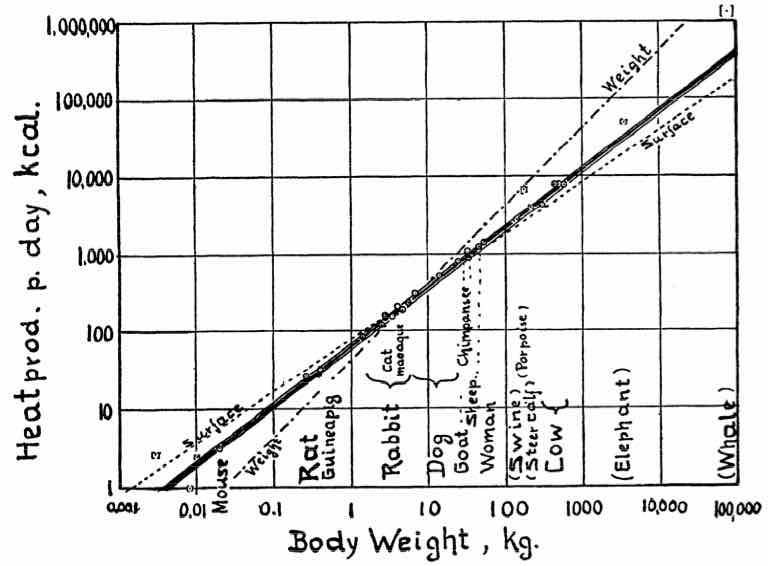## Moore’s Law

A idea originated around 1970, by George Moore from Intel

The simple version of this law states that processor speeds will double every two years

More specifically, it says that the number of transistors on an affordable CPU would double every two years

(see paper)## Intel says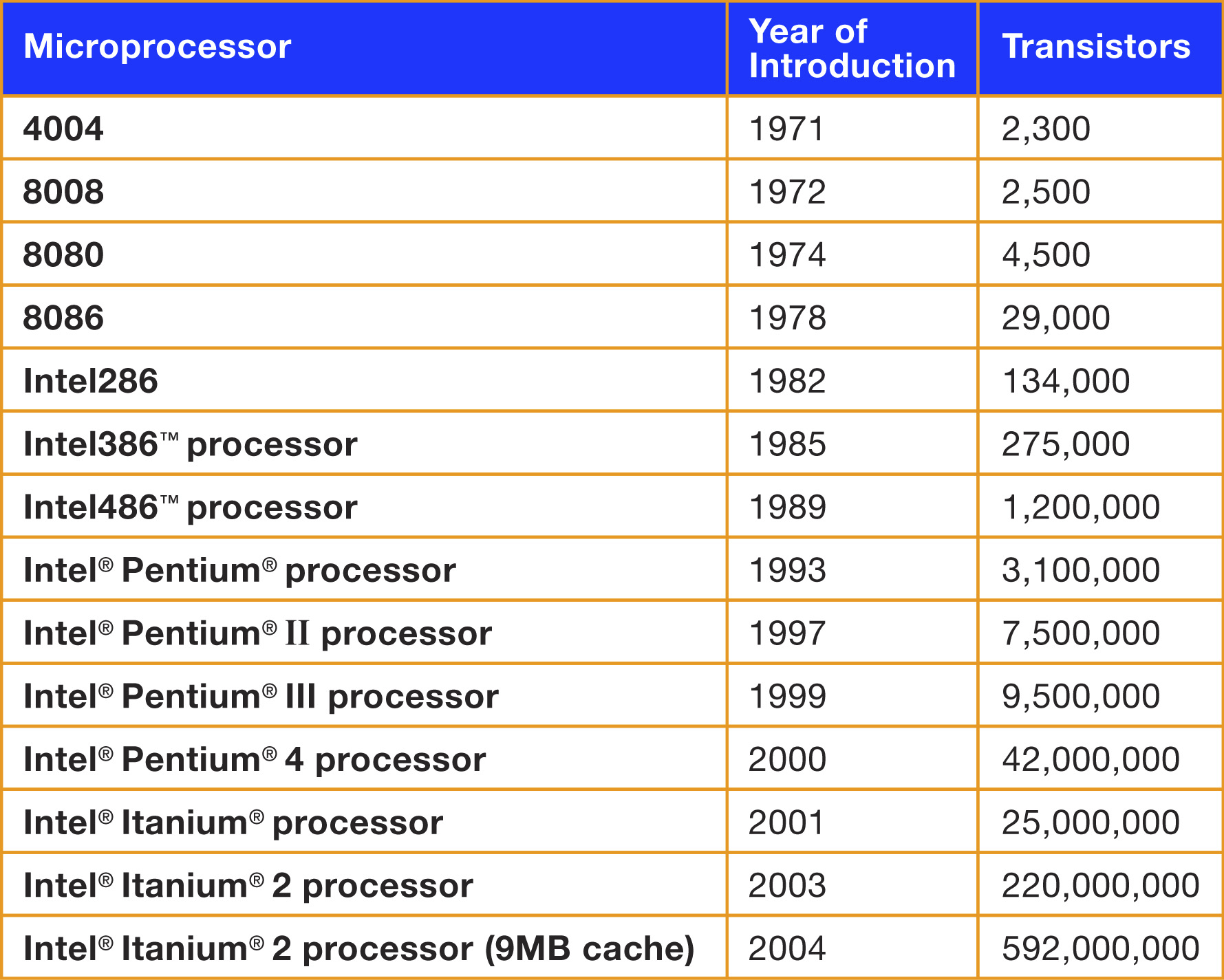## Real data: Number of transistors in chips v/s year

plot(count~Date, data=trans)## Semilog scale: Number of transistors v/s year

plot(log(count) ~ Date, data=trans)## Semi-log means exponential growth

we have straight line on the semi-log

That is, log(y) versus x $\log(y)=log(I)+log(R)\cdot x$ In this case the original relation is $y=I\cdot R^x$

## Model

model <- lm(log(count) ~ Date, data=trans)
exp(coef(model))
(Intercept)        Date
7.83e-295    1.41e+00 

## Semi-log scale and model

plot(count ~ Date, data=trans, log="y")
lines(exp(predict(model)) ~ Date, data=trans)## Meaning

Every year processors grow by a factor of

exp(coef(model))
Date
1.41 

## Same happens with DNA

### Cost of sequencing human genome## The Robots Are Coming

### John Lanchester

• 1992 Russo-American moratorium on nuclear testing
• 1996 Computer simulations to design new weapons
• Needed more computing power than could be delivered by any existing machine

## The Robots Are Coming

### John Lanchester

• USA designed ASCI Red, the first supercomputer doing over one teraflop
• ‘flop’ is a floating point operation (multiplication) per second
• teraflop is $$10^{12}$$ flops
• In 1997, ASCI Red did 1.8 teraflops
• The most powerful supercomputer in the world until about the end of 2000.

## The Robots Are Coming

In his book, John Lanchester says

"I was playing on Red only yesterday – I wasn’t really, but I did have a go on a machine that can process 1.8 teraflops.

"This Red equivalent is called the PS3: it was launched by Sony in 2005 and went on sale in 2006.

## The Robots Are Coming

"Red was [the size of] a tennis court, used as much electricity as 800 houses, and cost US\$55 million. The PS3 fits under the TV, runs off a normal power socket, and you can buy one for £200.

"[In 10 years], a computer able to process 1.8 teraflops went from being something that could only be made by the world’s richest government […], to something a teenager could expect [as a gift].